Maths-
General
Easy

Question

# The slope of the tangent to the curve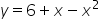at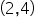is

## 10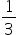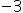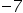Hint:

## The correct answer is:### The given curve is y = 6 + x + x2.We have to find the slope tangent at point (2,4)To find the slope we differentiate the curve w.r.t x.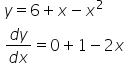Slope m =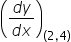m = (1 - 2 × 2)= 1 - 4= -3The slope of the tangent at given point is -3.

To find the slope we differentiate the given curve w.r.t x.#### With Turito Foundation.#### Get an Expert Advice From Turito.